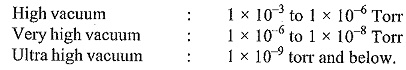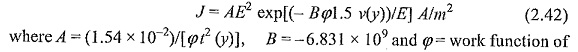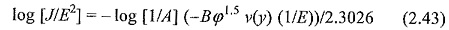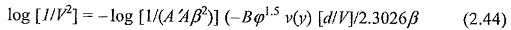## Vacuum Insulation:

The idea of using Vacuum Insulation purposes is very old. According to the Townsend theory, the growth of current in a gap depends on the drift of the charged particles. In the absence of any such particles, as in the case of perfect vacuum, there should be no conduction and the vacuum should be a perfect insulating medium. However, in practice, the presence of metallic electrodes and insulating surfaces within the vacuum complicate the issue and, therefore, even in vacuum, a sufficiently high voltage will cause a breakdown.

In recent years a considerable amount of work has been done to determine the electrical properties of high vacuum. This is mainly aimed at adopting such a medium for a wide range of applications in devices such as vacuum contactors and interrupters, high frequency capacitors and relays, electrostatic generators, microwave tubes, etc. The contactors and circuit breakers using vacuum as insulation are finding increasing applications in power systems.

### What is Vacuum?

A vacuum system which is used to create vacuum is a system in which the pressure is maintained at a value much below the atmospheric pressure. In vacuum systems the pressure is always measured in terms of millimetres of mercury, where one standard atmosphere is equal to 760 millimetres of mercury at a temperature of 0°C. The term “millimetres of mercury” has been standardized as “Torr” by the International Vacuum Society, where one millimetre of mercury is taken as equal to one Torr.

#### Vacuum Insulation may be classified asFor electrical insulation purposes, the range of vacuum generally used is the “high vacuum”, in the pressure range of  10–3 Torr to 10-6 Torr.

### Electron Emission in Vacuum

When the voltage across a small vacuum gap (<2 mm) is increased, a relatively small current, mainly due to electrons, flows. For higher gaps (< 10 mm) small pulses of current, called microdischarges flow either independently or superposed over the steady current. For both the types of gaps subsequent increase of applied voltage causes the breakdown of the gap.

Several mechanisms have been proposed to explain electron emission from metallic surfaces. The most widely accepted mechanism is the cold emission model originally proposed by Fowler and Nordheim. Accordingly to this, the current densityJdue to field emission from sharp points on the electrode is given as:The expression t(y) and ν(y) are slow varying functions which are regarded as constants. The above equation therefore can be expressed as:Since A, Φ and essentially ν(y) are constants, a plot of log J/E2 against the reciprocal of E produces a straight line having a negative slope. This straight line is often used to assess the applicability of Fowler-Nordheim relation of the experimental data. However, in the case of electrodes with large area, the protrusions and uneven surfaces become unknown geometry. Therefore, to take account of these factors, Alpert modified the above equation as followswhere A′ is the electrode area and d is the gap length, J has been replaced by (1/A′), and the modified field is written as a product of local field enhancement factor β and the average field E (= V/d). The actual emitting area is replaced as A′β2. Eqs. (2.42 to 2.44) have been experimentally confirmed by many investigators. If β is high and critical macroscopic field exceeds the field in the gap, the emitting site will explode releasing metal vapour.

In Vacuum Insulation, electrode surfaces in long gaps produce low power pulses called microdischarges. These discharges have durations of 0.1 to 100 ms, frequency of 0.1 to 100 Hz and amplitudes of ≤ 10 mA. They may be caused by small particles of electrode material that are pulled out from one electrode and strike the other, or the beam of electrons from a cathode that can vapourize a small quantity of electrode material. At a given pressure, the frequency of occurrence of microdischarges increase with increase in applied voltage, eventually leading to breakdown.

Scroll to Top